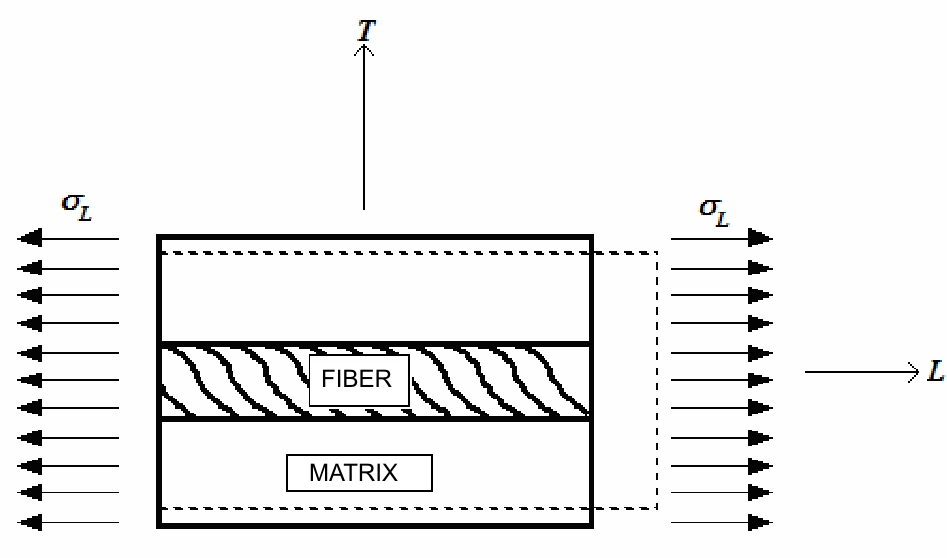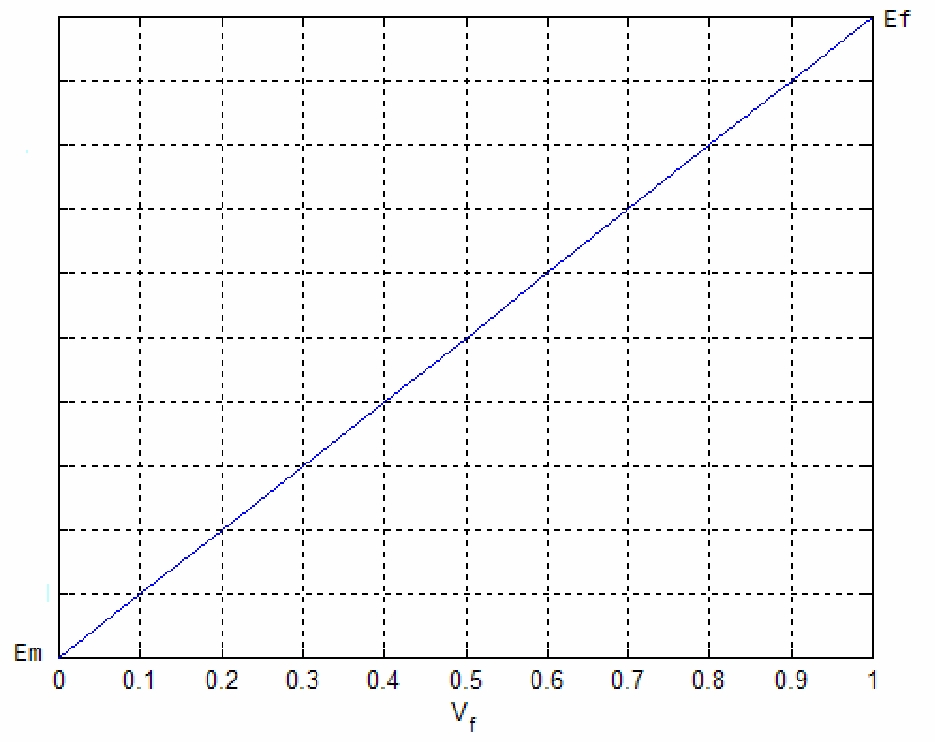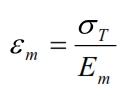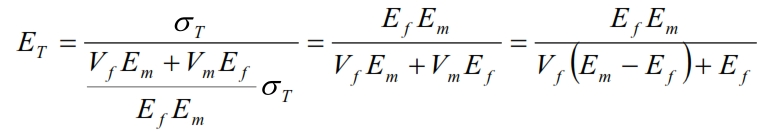﻿ Calculation of Elastic Constants | Aerospace Engineering

## 2.6. Calculation of Elastic Constants

>> Composite Analysis Tool

Consider an element of the laminate as depicted in Figure below, suppose that composite is subjected to deformation εL in the direction of the fibers. The fiber will be stressed by a tension:

σf= Ef  ·εL

while the matrix is subjected to a stress tension equal to:

σm= Em  ·εm

where Ef  and Em are respectively the Young module of the fibers and matrix.Unit volume of laminate loaded along the direction of the fibers (L)

The area section A of unit volume of laminate (ortogonal to L direction, see figure above) is subjected to stress tension σL in order to this equivalence is valid:

P=σf  ·Af = σf  ·Af + σm ·Am

Since σf= Ef  ·εL, then:This formula of Young module rapresents a linear variation of EL, from  Em to Ef, when Vf varies from 0 to 1 (see figure below).Trend of young moduLe EL as a function of percentage of fibers in the unit volume

Young’s module in the T direction can be considered considering the same fundamental volume element of the of the plate, loaded with a stress σT (Figure below).The deformation in the T direction for the matrix is:The deformation for the fiber is:The trasversal area where εL is applied is approximatively VfW, while for εm is VmW. The total deformation is:  εT ·W=Vf ·W·εL + Vm·W· εL è  εT =Vf ·εL + Vm· εL

Then using the previous formulas of εL and εT:Thus:By means can be calculated the Young module in the T direction:With similar considerations, we can derive the values of the Poisson coefficients and the tangential module: